Home > Excel > Tutorials > Shaded BandShare Your

Keywords: TQM, track deviation, shaded area (or zone) on x-y chart, colored zone, color zone, colour zone, coloured zone, unstacked area

This suggestion for using unstacked area chart series to create the bands shown in Figure 1 comes from Jon Peltier.  He is a regular contributor to the Excel newsgroups.   The basic idea is to use a combination chart, with unstacked area chart series for the +/- sigma bands on the primary axes, and XY Scatter for the data on the secondary axes.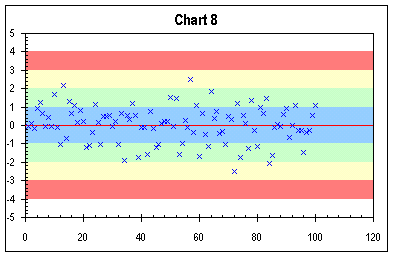Figure 1

The data points for plotting are in A2:A101, and the sigma band data are in C2:K3 as in Figure 2.  In this example, the data are randomly created using the =NORMSINV(RAND()) function.  Of course, in a real application, the data would come from an external source.  Similarly, in this case, the limits for the sigma bands are known to have the value -3, -2, ..., +3.  Alternatively, one could always calculate the limits from the mean and the standard deviation of the sample.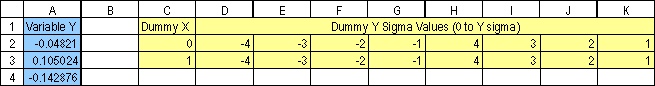Figure 2

Step 1 (Chart 1): Create a XY Scatter chart of the random data in A2:A101.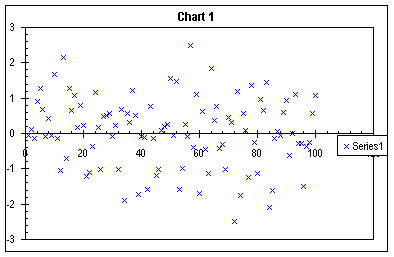Chart 1

Step 2 (Chart 2): Add the sigma data in C2:K3. This is with the copy/paste special method, adding new series, as columns, with the category values in the first column.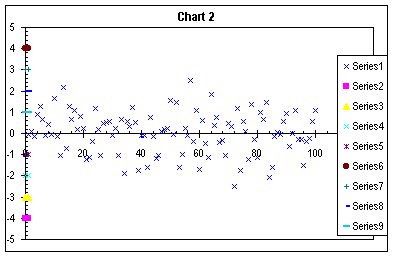Chart 2

Step 3 (Chart 3):  Assign Series 1 (the random data) to the secondary axes.Chart 3

Step 4 (Chart 4): Some intermediate housekeeping. Format the Secondary Y axis so that the Value X axis crosses at -5000 (an arbitrarily large number that assures the axis labels and ticks stay at the bottom). The primary X axis crosses the primary Y axis at zero. In Chart Options, add the secondary X axis.  While it is possible to remove the Secondary Y axis, leave it for clarity, and remove it later.Chart 4

Step 5 (Chart 5): Convert Series 2 to an Unstacked Area type series, the first of the area chart options.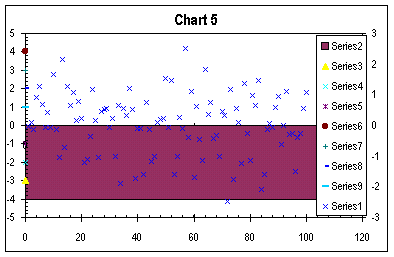Chart 5

Step 6 (Chart 6): Repeat the Unstacked Area conversion for Series 3 through 9. Luckily, the F4 key works to repeat chart type selection.Chart 6

Step 7 (Chart 7): Format the area series to indicate deviation from the mean. Series 2 and 6 are filled red for >3 sigma; series 3 and 7 are yellow for >2 sigma; series 4 and 8 are green for >1 sigma; and series 5 and 9 are blue for <1 sigma. In this example, the series have no border, but that's a user preference.Chart 7

Step 8 (Chart 8): Complete remaining housekeeping:

Delete the legend

In Chart Options finally remove that secondary Y axis; if it's left on the chart, the user must ensure that its scaling matches that of the primary Y axis.*

Format the primary category (X) axis to have no major or minor ticks and no labels.

In the example, the axis line is set to red to show the mean, but that's another user preference.Chart 8

* There are advantages to splitting the data and the sigma bands to primary and secondary axes. The major one is that as points are added, you do not need to expand the range of dummy points. A major disadvantage of having both Y axes is the need to ensure that both are identically scaled.